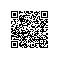# JavaScript---网络编程(4)-Date、Math、Global和自定义对象

function println(parma){
document.write(parma+"<br/>");
}
function print(parma){
document.write(parma);
}


# Date 对象

dateObj = new Date()
dateObj = new Date(dateVal)
dateVal

dateObj = new Date(year, month, date[, hours[, minutes[, seconds[,ms]]]])

Date 对象保存以毫秒为单位表示特定时间段。如果某个参数的值大于其范围或为负数，则存储的其他值将做相应的调整。例如，如果指定 150 秒，JScript 将该数字重新定义为 2 分 30 秒。

Date 对象能够表示的日期范围约等于 1970 年 1 月 1 日前后各 285,616 年。

Date 对象具有两个不创建 Date 对象就可以调用的静态方法。它们是 parse 和 UTC。

<html>
<head>
<title>Date对象的用法演示</title>
<meta http-equiv="Content-Type" content="text/html; charset=UTF-8" />
</head>
<body>
<script type="text/javascript" src="out.js">
</script>
<script type="text/javascript">
var date = new Date();//当前日期时间，它的精确值= 从1970.1.1开始，到现在经过的毫秒数
println(date);//自带toString()
println(date.toString());//默认是GMT格式---Mon Jun 06 2016 20:03:45 GMT+0800 (中国标准时间)
println(date.toDateString());//默认是GMT格式---Mon Jun 06 2016
println(date.toLocaleString());//本地格式---2016/6/6 下午8:05:48
println(date.toLocaleDateString());//本地格式---2016/6/6---只有日期
//综上，带Date的只显示日期
println("<hr/>");

var year = date.getFullYear();//获取现在的年份
var month = date.getMonth() + 1;//跟java一样，返回的月份和我们平时用的小1---它是序号-所以加一才是真正的月份
var day = date.getDate();//这才是我们要的日期---注意，getDay()返回的是星期几
var weekDay = date.getDay();//星期几
println(year + "-" + month + "-" + day + ",星期" + weekDay);
println(year + "-" + month + "-" + day + "," + getWeekDay(weekDay));

function getWeekDay(weekDay){
var weeks = ["星期日", "星期一", "星期二", "星期三", "星期四", "星期五", "星期六"];
return weeks[weekDay];
}

//日期对象和毫秒值的转换

var date2 = new Date();
date2.setFullYear(2015);//只更改年份
var time = date2.getTime();//日期-->毫秒值
println("time=" + time);
var date3 = new Date(time);//毫秒值-->日期
println(date3.toLocaleString());

//字符串跟日期之间的转换
//日期对象-->字符串 ： 直接用toLocalString()，toLocalDateString()
//字符串-->日期对象
var strDate = "6/4/2016";//格式: 月份/日期/年份

//var strDate = "6/4/19";//不要这么写
//格式: 月份/日期/年份
//IE ：如果年份只给2位则前面默认是19**
//360浏览器：如果年份只给2位则前面默认是20**---超过50就前面默认是19**
//年份值可以用 2 位数字表示也可以用 4 位数字表示。如果使用 2 位数字来表示年份，
//那么该年份必须大于或等于 70。

var time2 = Date.parse(strDate);//静态方法，返回值是毫秒值
println(time2);
var date4 = new Date(time2);
println(date4.toLocaleString());

</script>

<!-- with语句演示 -->
<script type="text/javascript">
//在with内部的方法调用时可以省略该date对象
with(date){
var year = getFullYear();
var month = getMonth()+1;//注意加一
var day = getDate();
println(year+"-"+month+"-"+day);
}
</script>

</body>
</html>


360浏览器8.1 演示结果：# Math 对象

Math.[{property | method}]

property

method

Math 对象不能用 new 运算符创建，如果试图这样做则给出错误。该对象在装载脚本引擎时由该引擎创建。其所有方法和属性在脚本中总是可用。

<html>
<head>
<title>Math对象的用法演示</title>
<meta http-equiv="content-type" content="text/html; charset=UTF-8">
</head>

<body>
<script type="text/javascript" src="out.js">
</script>

<script type="text/javascript">
var num1=Math.ceil(23.456);//返回大于等于其数字参数的最小整数。
var num2=Math.floor(23.456);//返回小于等于其数值参数的最大整数。
var num3=Math.pow(2,3);//表示2的3次方
println(num1+","+num2+","+num3);//24,23,8

var num4=Math.round(7.46);//四舍五入
println(num4);//7

//生成10个[0,10]范围内的随机整数
for(var x=0;x<10;x++){
var n=Math.random()*100;
if(n==99){
continue;
x--;
}
n=Math.floor(n/9);
println(n);
}

</script>

</body>
</html>


360浏览器8.1 演示结果：# Global 对象–异常以及for-in语句

Global 对象没有语法。直接调用其方法。

Global 对象从不直接使用，并且不能用 new 运算符创建。它在 Scripting 引擎被初始化时创建，并立即使其方法和属性可用。

<!DOCTYPE html>
<html>
<head>
<title>Global对象用法演示</title>
<meta http-equiv="content-type" content="text/html; charset=UTF-8">
</head>
<body>
<script type="text/javascript" src="out.js">
</script>
<script type="text/javascript">
/*
Global对象中的属性和方法，调用时可以省略：Global.
*/
println("123" + 4);//1234
//字符串输出

println(parseInt("123") + 4);//127
//数值相加输出

//注意，parseInt()这个方法和Java不同的地方：
//       1)不会抛异常(如果解析不了，它就返回NaN)
//       2)如果解析串中前面有数字，那么这部分前导数字会被单独解析出来当作整个方法的返回结果。但后面有数字则无法解析

println(parseInt("aaa123"));//NaN--Not a Number --非法的意思
println(parseInt("1234aaa"));//1234
println(parseInt("12345a1a1a123"));//12345
println("<hr/>");

//带进制的转换1： 把指定进制的格式串 转换成 十进制的整数
//---利用Global中的 parseInt(numString, [radix]) 方法
var num = parseInt("110", 10);//以十进制来解析
println(num);//110---输出10进制
var num = parseInt("110", 2);//以二进制来解析
println(num);//6---输出10进制
var num = parseInt("210", 2);//以二进制来解析
println(num);//NaN---代表非法---解析出错
var num = parseInt("210", 16);//以16进制来解析
println(num);//528
var num = parseInt("0x210", 16);//以16进制来解析
println(num);//528---和前面一样
//带进制的转换2：把 十进制的整数 转换成 其它进制的数
//---利用Number对象中的  toString([radix]) 方法

//var num = new Number(528);//和下面一样的
var num = 528;
println(num.toString(2)); //结果就是二进制的数： 1000010000
println(num.toString(16)); //结果就是16进制的数： 210
</script>
<!-- js中的异常处理及自定义异常 -->
<script type="text/javascript">
try {
// fun(1);//没有异常
fun(0);//有异常(自己抛出的异常)
}
catch (e) {
println(e);
}
finally {
println("bbbb");//不管有没有异常，都会执行
}

function fun(n){
if (n == 0) {
throw "exception 0";//相当于java中的手动抛自定义异常
}
}
</script>
<!--  for-in语句  -->
<script type="text/javascript">
/*
for( 变量名  in 对象 ){
...//分别对对象中的元素(属性)进行遍历操作
}
*/
//数组对象

var arr = [1,2,3,56,435,3];
for( x in arr){//注意，对于数组，这里的x是下标即是0,1,2,3,...
println( x+":  "+ arr[x] );
}

//用for...in语句操作自定义对象---见后面部分的代码演示

</script>
</body>
</html>


360浏览器8.1 演示结果：# 自定义对象-for-in语句

<html>
<head>
<title>自定义对象的用法演示</title>
<meta http-equiv="content-type" content="text/html; charset=UTF-8">
</head>
<body>
<script type="text/javascript" src="out.js">
</script>
<!-- 用js来描述我们自己的对象，如类似java当中的Person类 -->
<script type="text/javascript">
/*  js是基于对象的，如果要描述对象，那么可以用function。因为js中的
function功能和java中的类(模板) 本质上是差不多的---里面可以添加变量，也可以添加自定义函数
*/
function Person(){
//alert("Person的构造方法...");
}

var p = new Person();
//给对象添加属性，直接采用“p.属性名=”的形式赋值就行---如果没有则是添加，如果有则是修改
//※※方式1：在对象外面添加属性和方法
p.name = "Jack";
p.age = 23;
//alert(p.name+","+p.age);
//给对象添加函数
p.info = function(){//相当于toString()
return this.name + "," + this.age;
};

println("p的信息:" + p.info());
</script>
<script type="text/javascript">
var obj = new Object();
//※※方式1：在对象外面添加属性和方法
//1给对象添加属性
obj.name = "god";
obj.age = 10000;

//2给对象添加属性
obj.toString = function(){
return this.name + "," + this.age;
}
println(obj);
</script>
<!--
注意：前面用“对象.prototype.属性或函数名=***”的形式，更改的是原型对象
而本例用 “对象.属性或函数名=***”的形式，更改的是当前对象(原型对象的克隆体)
-->
<script type="text/javascript">
//js自定义对象的构造器+属性+方法
function Person(name, age){
//※※方式2：在函数对象里面添加属性和方法
this.name = name;
this.age = age;

this.toString = function(){
return this.name + "," + this.age;
};

this.setName = function(name){
this.name = name;
};
this.getName = function(){
return this.name;
};
}

var p2 = new Person("Tom", 22);
println("p2.name=" + p2.name + ", p2.age=" + p2.age);
println("p2= " + p2);
p2.setName("Rose");
println("p2=" + p2);
println("p2.getName=" + p2.getName());
</script>

<script type="text/javascript">
println("<hr/>");

//用for...in语句操作自定义对象
for( var x in p2){//x是函数中的成员变量与成员方法的名称
println("x="+x+"-----"+p2[x]);// p2[x]就是valeOf(x)
}

</script>

</body>
</html>


360浏览器8.1 演示结果：使用钉钉扫一扫加入圈子
+ 订阅